## How to Stack Data from Multiple Columns into One Column in Excel

Are you tired of having your data spread out across multiple columns, making it difficult to analyze and understand? It can be overwhelming to have to constantly switch back and forth between columns, hunting for the information you need. This post will show you how to stack data from multiple columns to one column in Microsoft Excel Spreadsheet.

This article will introduce you the two methods. After reading the article below, you will find the two ways are verify simple and convenient to operate in excel.

In previous article, I have shown you the method to split data from one long column to multiple columns by VBA and Index function.

For example, see the initial table below:

And we want reverse data into one column and make it looks like:

Now we can follow below two methods to make it possible. Let’s start it.

## 1. Stack Data in Multiple Columns into One Column by Formula

Step 1: Select range A1 to F2 (you want to do stack), in Name Box, enter a valid name like Range, then click Enter.

Step 2: In any cell you want to locate the first cell of destination column, enter the formula

``=INDEX(Range,1+INT((ROW(A1)-1)/COLUMNS(Range)),MOD(ROW(A1)-1+COLUMNS(Range),COLUMNS(Range))+1).``

Please be aware that you have to replace ‘Range’ in this formula to your defined name in Name Box.

Step 3: Click Enter. Verify that ‘ID’ (the value in the first cell of selected range) is displayed properly.

Step 4: Drag the fill handle to fill I column. Verify that data in previous initial location is reversed to one column properly.

Note:

If you drag the fill handle to cells extend selected range cell number, error will be displayed in redundant cell.

## 2. Stack Data in Multiple Columns into One Column by VBA

Step 1: On current visible worksheet, right click on sheet name tab to load Sheet management menu. Select View Code, Microsoft Visual Basic for Applications window pops up.

Or you can enter Microsoft Visual Basic for Applications window via Developer->Visual Basic.

Step 2: In Microsoft Visual Basic for Applications window, click Insert->Module, enter below code in Module1:

```Sub StackDataToOneColumn()
Dim Rng1 As Range, Rng2 As Range, Rng As Range
Dim RowIndex As Integer

Set Rng1 = Application.Selection
Set Rng1 = Application.InputBox("Select Range:", "StackDataToOneColumn",        Rng1.Address, Type:=8)
Set Rng2 = Application.InputBox("Destination Column:", "StackDataToOneColumn", Type:=8)

RowIndex = 0
Application.ScreenUpdating = False

For Each Rng In Rng1.Rows
Rng.Copy
Rng2.Offset(RowIndex, 0).PasteSpecial Paste:=xlPasteAll, Transpose:=True
RowIndex = RowIndex + Rng.Columns.Count
Next
Application.CutCopyMode = False
Application.ScreenUpdating = True
End Sub```

Step 3: Save the codes, see screenshot below. And then quit Microsoft Visual Basic for Applications.

Step 4: Click Developer->Macros to run Macro. Select ‘StackDataToOneColumn’ and click Run.

Step 5: Stack Data to One Column dialog pops up. Enter Select Range \$A\$1:\$F\$2. Click OK. In this step you can select the range you want to do stack.

Step 6: On Stack Data to One Column, enter Destination Column \$I\$1. Click OK. In this step you can select the first cell from destination range you want to save data.

Step 7: Click OK and check the result. Verify that data is displayed in one column properly. The behavior is as same as the result in method 1 step# 4.

## 3. Merge Cells into One in Excel

To merge or consolidate cells in Microsoft Excel, follow these steps:

Step 1: Select the cells you want to merge.

Step 2: Right-click on the selected cells and choose “`Merge Cells`” from the context menu.

Step 3: Alternatively, you can click on the “`Merge & Center`” button in the “`Alignment`” section of the “`Home`” tab on the ribbon.

## 4. Combine two columns in excel without losing data

To combine two columns in Microsoft Excel without losing data, you can use the following formula:

Step 1: In a new column C1, enter the following formula:

``=A1 & B1``

If you want to combine data from two or multiple cells in Excel, you can also use this formula.

Step 2: Press Enter to apply the formula.

Step 3: Drag the formula down to the end of the data in the columns.

The formula uses the & operator to concatenate the contents of two cells. In this example, the formula combines the contents of cells A2 and B2 into a single cell. By dragging the formula down, you can combine the contents of all the cells in the two columns.

You can also use the CONCATENATE function to combine columns, which works in a similar manner. The formula would look like this:

``=CONCATENATE(A1, B1).``

## 5. Combine 2 Columns with a Space

If you want to combine two columns with a space between them, you can use the following formula:

``=A1 & " " & B1``

In this example, the formula combines the contents of cells A1 and B1 into a single cell, separated by a space. By dragging the formula down, you can combine the contents of all the cells in the two columns.

## 6. Append Columns in Excel

If you want to append two columns in Microsoft Excel, just follow these steps:

Step 1: Select the first column of data that you want to append.

Step 2: Right-click on the selected cells and choose “`Copy`” from the context menu.

Step 3: Select the second column of data that you want to append, and right-click on the first cell in the column.

Step 4: Choose “`Insert Copied Cells`” from the context menu.

This will insert a copy of the first column of data after the second column, effectively appending the two columns.

## 7. Conclusion

Stacking data from multiple columns into one is a useful operation in Microsoft Excel when you need to combine and analyze data from different sources. The process can be easily achieved by using the built-in functions such as the & operator or the CONCATENATE function, which allow you to concatenate the contents of multiple cells into one.

Hope you can like this post.

## 8. Related Functions

• Excel INDEX function
The Excel INDEX function returns a value from a table based on the index (row number and column number)The INDEX function is a build-in function in Microsoft Excel and it is categorized as a Lookup and Reference Function.The syntax of the INDEX function is as below:= INDEX (array, row_num,[column_num])…
• Excel ROW function
The Excel ROW function returns the row number of a cell reference.The ROW function is a build-in function in Microsoft Excel and it is categorized as a Lookup and Reference Function.The syntax of the ROW function is as below:= ROW ([reference])….
• Excel MOD function
he Excel MOD function returns the remainder of two numbers after division. So you can use the MOD function to get the remainder after a number is divided by a divisor in Excel. The syntax of the MOD function is as below:=MOD (number, divisor)….
• Excel INT function
The Excel INT function returns the integer portion of a given number. And it will rounds a given number down to the nearest integer.The syntax of the INT function is as below:= INT (number)…
• Excel COLUMNS function
The Excel COLUMNS function returns the number of columns in an Array or a reference.The syntax of the COLUMNS function is as below:=COLUMNS (array)….

## How to Split Word into Different Cells in Excel

This post will guide you how to split word into different columns with a formula in Excel. How do I split word or number into separate cells with VBA Macro code in Excel 2010/1013/2016.

## Split Word into Different Cells with Formula

Assuming that you have a list of data in range B1:B4, and you need to split text value or a word into separate cells in your worksheet. How to do it. You can use a formula based on the MID function and the COLUMNS function. Like this:

`=MID(\$B1,COLUMNS(\$B\$1:B\$1),1)`

Type this formula in cell C1 and press Enter key, and then drag the AutoFill Handle over to other cells in Row1.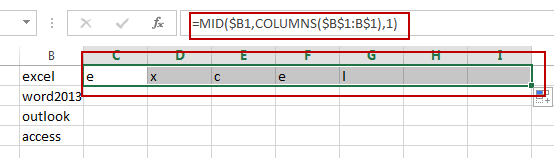Then you still need to drag the AutoFill Handle down to other cell to apply this formula for B2:B4.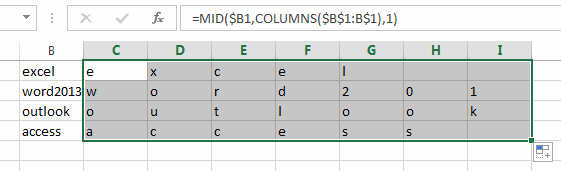## Split Word into Different Cells with VBA Macro

You can also use an Excel VBA Macro code to achieve the same result of splitting word into different cells. Here are the steps:

#1 open your excel workbook and then click on “Visual Basic” command under DEVELOPER Tab, or just press “ALT+F11” shortcut.#2 then the “Visual Basic Editor” window will appear.

#3 click “Insert” ->”Module” to create a new module.#4 paste the below VBA code into the code window. Then clicking “Save” button.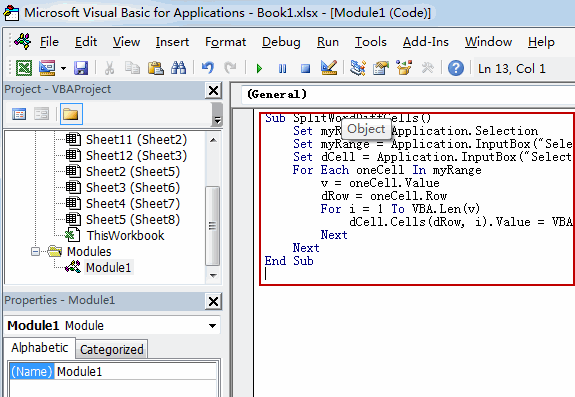#5 back to the current worksheet, then run the above excel macro. Click Run button.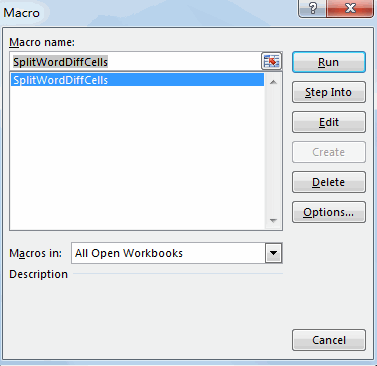#6 Select one Range that you want to split words. Click Ok button.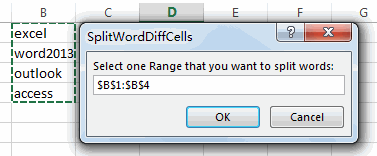#7 Select one destination Cell that you want to place. Click Ok button.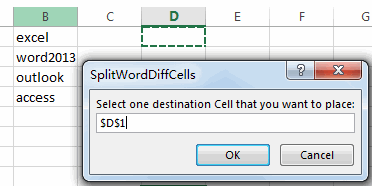#8 Let’see the result:### Related Functions

• Excel MID function
The Excel MID function returns a substring from a text string at the position that you specify.The syntax of the MID function is as below:= MID (text, start_num, num_chars)…
• Excel COLUMNS function
The Excel COLUMNS function returns the number of columns in an Array or a reference.The syntax of the COLUMNS function is as below:=COLUMNS (array)….

## Split Data in One Column to Multiple Columns

This post will guide you how to split data in a single column into multiple columns with a formula in Excel. How do I transpose data from one column to multiple columns in Excel. How can I make one long column into multiple columns in Excel.

## Split Data in One Column to Multiple Columns

Assuming that you have 5 sets of product’s list in one column, and you would like to convert each set in its own column or split each sets into its own staked columns. How to achieve it.

If you want to split each sets in one column from row to row in stacked columns, and you need to create a formula based on the OFFSET function, the COLUMNS function, and the ROWS function.

Just like this:

`=OFFSET(\$A\$1,COLUMNS(\$A1:A1)-1+(ROWS(\$1:1)-1)*2,0)`

Type this formula into a blank cell C1, and press Enter key in your keyboard, and then drag the AutoFill Handle from C1 to D1. Select the Cell C1 and D1, then drag the AutoFill Handle in Cell D1 from D1 to D5.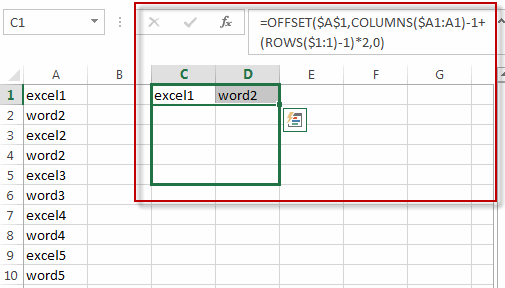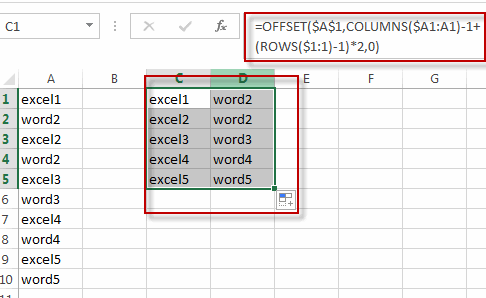If you want to split each sets in one column from one column into another column, you just need to use the following formula based on the INDEX function, the ROW function and the COLUMNS function. Like this:

`=INDEX(\$A\$1:\$A\$10,ROW(C1)+(2*(COLUMNS(\$C\$1:C\$1)-1)))`

Type this formula into the formula box of the Cell C1, and press Enter key in your keyboard, and drag the AutoFill Handle from C1 to C2. Select the Cell C1 and C2, and then drag the AutoFill handle in Cell C2 from C2 to G2.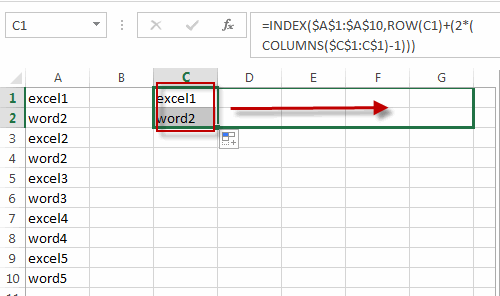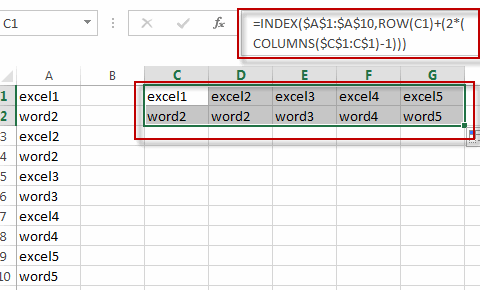### Related Functions

• Excel INDEX function
The Excel INDEX function returns a value from a table based on the index (row number and column number)The INDEX function is a build-in function in Microsoft Excel and it is categorized as a Lookup and Reference Function.The syntax of the INDEX function is as below:= INDEX (array, row_num,[column_num])…
• Excel ROW function
The Excel ROW function returns the row number of a cell reference.The ROW function is a build-in function in Microsoft Excel and it is categorized as a Lookup and Reference Function.The syntax of the ROW function is as below:= ROW ([reference])….
• Excel ROWS function
The Excel ROWS function returns the number of rows in a cell reference.The ROWS function is a build-in function in Microsoft Excel and it is categorized as a Lookup and Reference Function.The syntax of the ROWS function is as below:= ROWS(array)….
• Excel COLUMNS function
The Excel COLUMNS function returns the number of columns in an Array or a reference.The syntax of the COLUMNS function is as below:=COLUMNS (array)….

## Sum Specific Row or Column in Named Range

This post will guide you how to sum a specific Row or Column in a named range in excel. Normally, you can easily sum a certain row or column in a worksheet in excel. But if you just want to sum a particular column or row in a named range, how to achieve it in excel.

## Sum Specific Row in Named Range

Assuming that you have a name range “excelhow”, if you want to sum a specific row (row 2) in named range, you need to create a formula based on the SUM function and the OFFSET function and the COLUMNS function to achieve the reuslt. Just like this:

`=SUM(OFFSET(excelhow,1,0,1,COLUMNS(excelhow)))`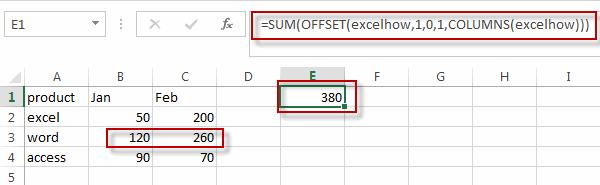Type this formula into a blank cell and then press enter key in your keyboard, you will see that the second row in the named range has been summed.

If you want to sum the first row in the named range “excelhow”, you just need to change the first “1” number as “0”. Just like the below formula:

`=SUM(OFFSET(excelhow,0,0,1,COLUMNS(excelhow)))`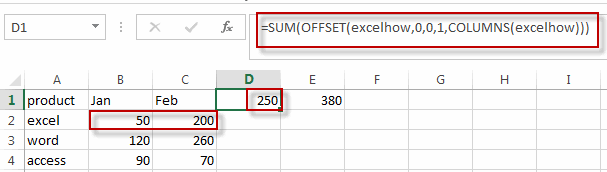## Sum Specific Column in Named Range

If you want to sum a specific column (column1) in the named range “excelhow”, you need to create a formula with the SUM function in combination with the INDEX function. Just like this:

`=SUM(INDEX(excelhow,,1))`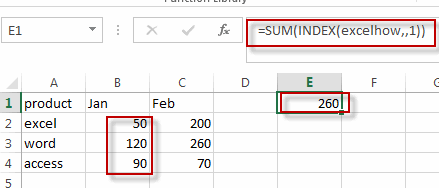If you want to sum the second column in the named range “excelhow”, you just need to change the number “1” in this formula as “2”.

`=SUM(INDEX(excelhow,,2))`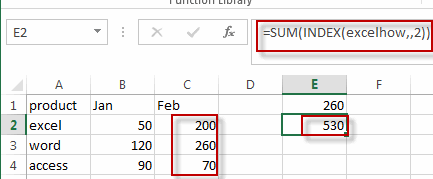### Related Functions

• Excel SUM function
The Excel SUM function will adds all numbers in a range of cells and returns the sum of these values. You can add individual values, cell references or ranges in excel.The syntax of the SUM function is as below:= SUM(number1,[number2],…)…
• Excel INDEX function
The Excel INDEX function returns a value from a table based on the index (row number and column number)The INDEX function is a build-in function in Microsoft Excel and it is categorized as a Lookup and Reference Function.The syntax of the INDEX function is as below:= INDEX (array, row_num,[column_num])…
• Excel Columns function
The Excel COLUMNS function returns the number of columns in an Array or a reference.The syntax of the COLUMNS function is as below:=COLUMNS (array)….

## Transpose Multiple Columns into One Column

This post will guide you how to transpose multiple columns into a single columns with multiple rows in Excel. How do I put data from multiple columns into one column with Excel formula; How to transpose columns into single column with VBA macro in excel.

Assuming that you have a data list in range B1:D4 contain 3 columns and you want to transpose them into a single column F. just following the below two ways.

## Transpose Multiple Columns into One Column with Formula

You can use the following excel formula to transpose multiple columns that contain a range of data into a single column F:

#1 type the following formula in the formula box of cell F1, then press enter key.

`=INDEX(\$B\$1:\$D\$4,1+INT((ROW(B1)-1)/COLUMNS(\$B\$1:\$D\$4)),MOD(ROW(B1)-1+COLUMNS(\$B\$1:\$D\$4),COLUMNS(\$B\$1:\$D4))+1)`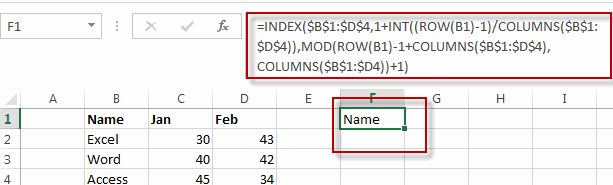#2 select cell F1, then drag the Auto Fill Handler over other cells until all values in range B1:D4 are displayed.

#3 you will see that all the data in range B1:D4 has been transposed into single column F.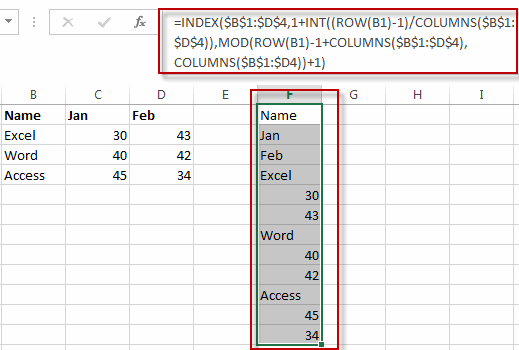## Transpose Multiple Columns into One Column with VBA Macro

You can also write an Excel VBA Macro to transpose the data of range in B1:D4 into single column F quickly. Just do the following steps:

#1 click on “Visual Basic” command under DEVELOPER Tab.#2 then the “Visual Basic Editor” window will appear.

#3 click “Insert” ->”Module” to create a new module.#4 paste the below VBA code into the code window. Then clicking “Save” button.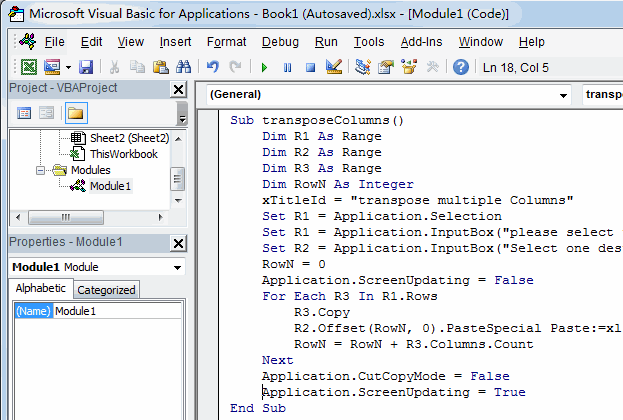```Sub transposeColumns()
Dim R1 As Range
Dim R2 As Range
Dim R3 As Range
Dim RowN As Integer
wTitle = "transpose multiple Columns"
Set R1 = Application.Selection
Set R1 = Application.InputBox("please select the Source data of Ranges:", wTitle, R1.Address, Type:=8)
Set R2 = Application.InputBox("Select one destination single Cell or column:", wTitle, Type:=8)
RowN = 0
Application.ScreenUpdating = False
For Each R3 In R1.Rows
R3.Copy
R2.Offset(RowN, 0).PasteSpecial Paste:=xlPasteAll, Transpose:=True
RowN = RowN + R3.Columns.Count
Next
Application.CutCopyMode = False
Application.ScreenUpdating = True
End Sub```

#5 back to the current worksheet, then run the above excel macro. Click Run button.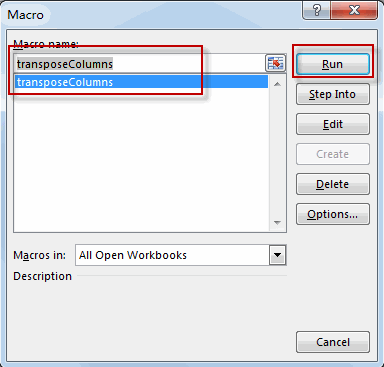#6 select the source data of ranges, such as: B1:D4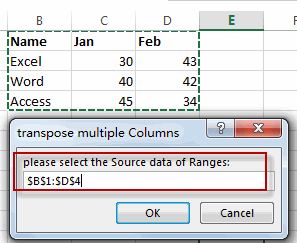#7 select one single cell in the destination Column, such as: F1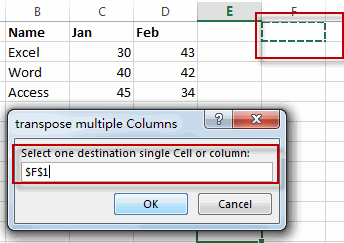#8 let’s see the last result.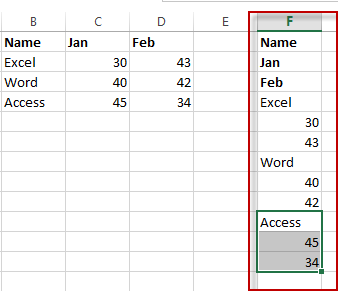### Related Functions

• Excel INDEX function
The Excel INDEX function returns a value from a table based on the index (row number and column number)The INDEX function is a build-in function in Microsoft Excel and it is categorized as a Lookup and Reference Function.The syntax of the INDEX function is as below:= INDEX (array, row_num,[column_num])…
• Excel INT function
The Excel INT function returns the integer portion of a given number. And it will rounds a given number down to the nearest integer.  And the INT function rounds down, so if you provide a negative number, the returned value will become more negative.The syntax of the INT function is as below:= INT (number)…
• Excel ROW function
The Excel ROW function returns the row number of a cell reference.The ROW function is a build-in function in Microsoft Excel and it is categorized as a Lookup and Reference Function.The syntax of the ROW function is as below:= ROW ([reference])….
• Excel Columns function
The Excel COLUMNS function returns the number of columns in an Array or a reference.The syntax of the COLUMNS function is as below:=COLUMNS (array)….
• Excel MOD function
he Excel MOD function returns the remainder of two numbers after division. So you can use the MOD function to get the remainder after a number is divided by a divisor in Excel. The syntax of the MOD function is as below:=MOD (number, divisor)….

## How to Extract multiple match Values into different Columns or Rows

This post explains that how to extract multiple match values from a range, then put the values into the different columns in excel. And how to extract multiple matches into the different rows.

In the previous post, we talked that how to get the position of the nth occurrence of a value in a column, and this pose will refer to it to extract all matched values firstly, then put all returned values into the different columns.

## Extract multiple match values into separate columns

If you want to fetch all matches from a range then put it into cells in different columns, you can use a combination with the INDEX function, the SMALL function, the IF function,  the ROW function and the COLUMNS function to create a new excel formula.

For example, if you want to get all member names belong to “excel” team in the range B3:D7, then separate it into the different columns, such as: E2, F2…you can use the following formula:

`=IFERROR(INDEX(\$D\$3:\$D\$7,SMALL(IF(\$C\$3:\$C\$7="excel",ROW(\$C\$3:\$C\$7)-ROW(INDEX(\$C\$3:\$C\$7,1,1))+1),COLUMNS(\$E\$2:E2))),"")`

Let’s see how the above formula works:

= ROW(\$C\$3:\$C\$7)-ROW(INDEX(\$C\$3:\$C\$7,1,1))+1

The ROW function returns the relative position of all rows in the range C3:C7.  The returned result is another array.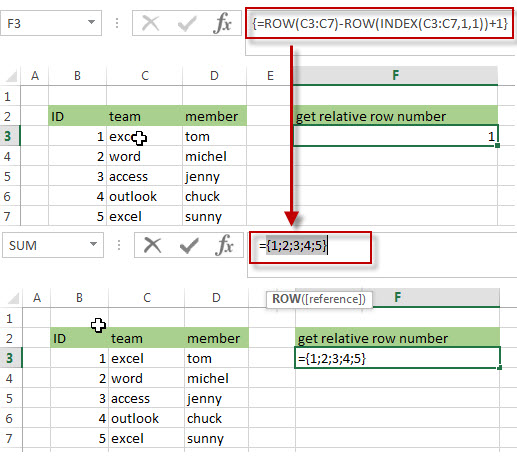= IF(\$C\$3:\$C\$7=”excel”,ROW(\$C\$3:\$C\$7)-ROW(INDEX(\$C\$3:\$C\$7,1,1))+1)

The IF function will check if each value in range C3:C7 is equal to “excel”, if TRUE, returns its relative position, otherwise, returns FALSE. As this formula is an array formula, so it must be returned an array result.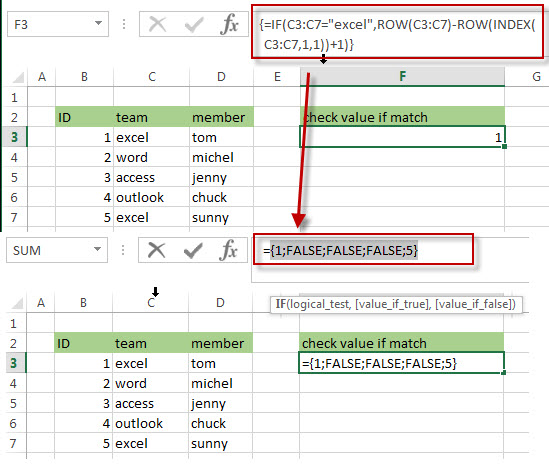=COLUMNS(\$E\$2:E2)

The COLUMNS function returns a numeric values, it will be used by the SMALL function as its nth argument to indicate the position of the number that you want to return.

=SMALL(IF(\$C\$3:\$C\$7=”excel”,ROW(\$C\$3:\$C\$7)-ROW(INDEX(\$C\$3:\$C\$7,1,1))+1),COLUMNS(\$E\$2:E2))

The SMALL function returns the position of the nth smallest values from the numbers in an array result returned by the above IF function. The nth argument is specified by the result returned by the COLUMNS function.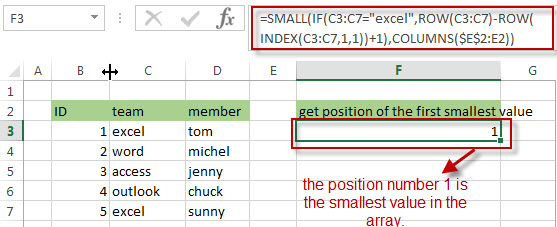=INDEX(\$D\$3:\$D\$7,SMALL(IF(\$C\$3:\$C\$7=”excel”,ROW(\$C\$3:\$C\$7)-ROW(INDEX(\$C\$3:\$C\$7,1,1))+1),COLUMNS(\$E\$2:E2)))

The INDEX function returns a value at a position returned by the above SMALL function in the range D3:D7, so it should be the first matches of the member name that belong to the excel team in the range B3:D7.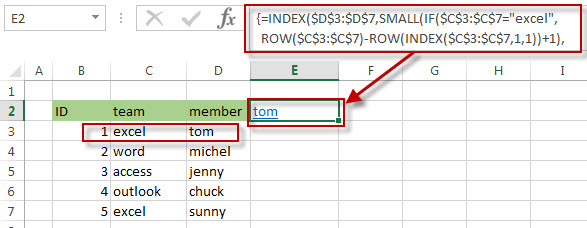And if you want to get the second matches into Cell F2, you just need to drag the fill hander in the Cell E2 to F2, then the second matches of the member name will be fetched in the Cell F2.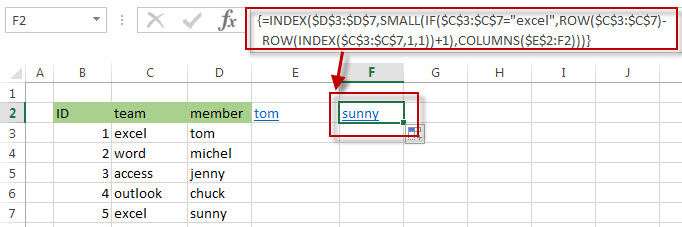=IFERROR()

The IFERROR function will check if the result returned by the SMALL function is a #NUM error, then returns an empty string.

## Extract multiple match values into separate rows

If you want to extract multiple matches into the separate rows in excel, just need to use ROWS instead of the COLUMNS function in the above formula as follows:

`=IFERROR(INDEX(\$D\$3:\$D\$7,SMALL(IF(\$C\$3:\$C\$7="excel",ROW(\$C\$3:\$C\$7)-ROW(INDEX(\$C\$3:\$C\$7,1,1))+1), ROWS(\$E\$2:E2))),"")`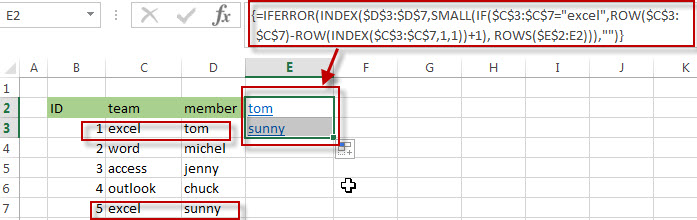If you want to extract the second matches, then put the result into the cell E3, you just need to drag the Fill Handler in Cell E2 to E3. The second matches of the member name should be fetched in the Cell E3.

### Related Formulas

• Get the Position of the nth Occurrence of a Character in a Cell
If you want to get the position of the nth occurrence of a character using a excel formula, you can use the FIND function in combination with the SUBSTITUTE function.
•  Get the position of Last Occurrence of a character or string in a cell
If you want to get the position of the last occurrence of a character in a cell, then you can use a combination of the LOOKUP function, the MID function, the ROW function, the INDIRECT function and the LEN function to create an excel formula.…
• Find the Relative Position in a Range or Table
If you want to know the relative row position for all rows in an Excel Range (B3:D6), you can use a excel Array formula as follows:=ROW(B3:D6)- ROW(B3) + 1. You can also use another excel array formula to get the same result as follows:=ROW(B3:D6)-ROW(INDEX(B3:D6,1,1))+1…
• Get the First Row Number in a Range
If the ROW function use a Range as its argument, it only returns the first row number.You can also use the ROW function within the MIN function to get the first row number in a range. You can also use the INDEX function to get the reference of the first row in a range, then combined to the ROW function to get the first row number of a range.…
•  Get the Last Row Number in a Range
If you want to get the last row number in a range, you need to know the first row number and the total rows number of a range, then perform the addition operation, then subtract 1, the last result is the last row number for that range.…
• Get the position of Last Occurrence of a value in a column
If you want to find the position number of the last occurrence of a specific value in a column (a single range), you can use an array formula with a combination of the MAX function, IF function, ROW function and INDEX Function.

### Related Functions

• Excel IFERROR function
The Excel IFERROR function returns an alternate value you specify if a formula results in an error, or returns the result of the formula.The syntax of the IFERROR function is as below:= IFERROR (value, value_if_error)…
• Excel SMALL function
The Excel SMALL function returns the smallest numeric value from the numbers that you provided. Or returns the smallest value in the array.The syntax of the SMALL function is as below:=SMALL(array,nth) …
• Excel INDEX function
The Excel INDEX function returns a value from a table based on the index (row number and column number)The INDEX function is a build-in function in Microsoft Excel and it is categorized as a Lookup and Reference Function.The syntax of the INDEX function is as below:= INDEX (array, row_num,[column_num])…
• Excel ROW function
The Excel ROW function returns the row number of a cell reference.The ROW function is a build-in function in Microsoft Excel and it is categorized as a Lookup and Reference Function.The syntax of the ROW function is as below:= ROW ([reference])….
• Excel MIN function
The Excel MIN function returns the smallest numeric value from the numbers that you provided. Or returns the smallest value in the array.The MIN function is a build-in function in Microsoft Excel and it is categorized as a Statistical Function.The syntax of the MIN function is as below:= MIN(num1,[num2,…numn])….
• Excel IF function
The Excel IF function perform a logical test to return one value if the condition is TRUE and return another value if the condition is FALSE. The IF function is a build-in function in Microsoft Excel and it is categorized as a Logical Function.The syntax of the IF function is as below:= IF (condition, [true_value], [false_value])….
• Excel ROWS function
The Excel ROWS function returns the number of rows in a cell reference.The ROWS function is a build-in function in Microsoft Excel and it is categorized as a Lookup and Reference Function.The syntax of the ROWS function is as below:= ROWS(array)….
• Excel COLUMNS function
The Excel COLUMNS function returns the number of columns in an Array or a reference.The syntax of the COLUMNS function is as below:=COLUMNS (array)….

## Excel Columns Function

This post will guide you how to use Excel COLUMNS function with syntax and examples in Microsoft excel.

### Description

The Excel COLUMNS function returns the number of columns in an Array or a reference.

The COLUMNS function is a build-in function in Microsoft Excel and it is categorized as a Lookup and Reference Function.

The COLUMNS function is available in Excel 2016, Excel 2013, Excel 2010, Excel 2007, Excel 2003, Excel XP, Excel 2000, Excel 2011 for Mac.

### Syntax

The syntax of the COLUMNS function is as below:

`=COLUMNS (array)`

Where the COLUMNS function arguments is:

• Array -This is a required argument.  A reference to a cell or a range of cells.

### Example

The below examples will show you how to use Excel COLUMNS Lookup and Reference Function to return the number of columns in a given range.

#1 To get the number of columns in the reference D1:F5, just using the following excel formula:

`=COLUMNS(D1:F5)`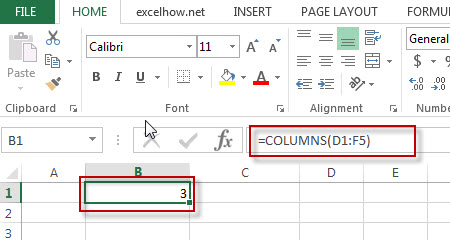### More Excel COLUMNS Formula Examples

• Transpose Multiple Columns into One Column
You can use the following excel formula to transpose multiple columns that contain a range of data into a single column, and you can also write an Excel VBA Macro to transpose the data of range in B1:D4 into single column F quickly…
• Sum Specific Row or Column in Named Range
Assuming that you have a name range “excelhow”, if you want to sum a specific row (row 2) in named range, you need to create a formula based on the SUM function and the OFFSET function and the COLUMNS function to achieve the reuslt….
• Split Data in One Column to Multiple Columns
If you want to split each sets in one column from row to row in stacked columns, and you need to create a formula based on the OFFSET function, the COLUMNS function, and the ROWS function..…